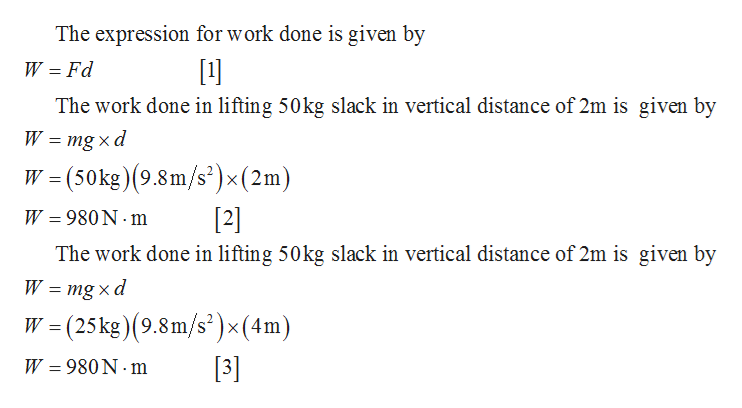# Which requires more work - lifting a 50-kg sack a vertical distance of 2m or lifting a 25-kg sack a vertical distance of 4m?

Question
78 views

Which requires more work - lifting a 50-kg sack a vertical distance of 2m or lifting a 25-kg sack a vertical distance of 4m?

check_circle

Step 1help_outlineImage TranscriptioncloseThe expression for work done is given by W = Fd  The work done in lifting 50kg slack in vertical distance of 2m is given by W = mg x d W = (50kg)(9.8m/s²)×(2m) W = 980 N · m  The work done in lifting 50kg slack in vertical distance of 2m is given by W = mg x d W = (25kg)(9.8m/s²)× (4m) W = 980 N · m  fullscreen

### Want to see the full answer?

See Solution

#### Want to see this answer and more?

Solutions are written by subject experts who are available 24/7. Questions are typically answered within 1 hour.*

See Solution
*Response times may vary by subject and question.
Tagged in